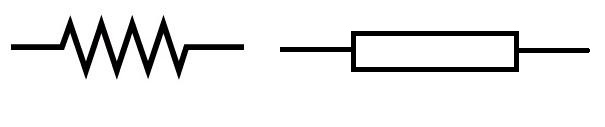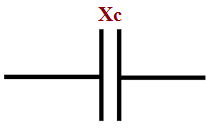# 07 Simple Difference between Resistance and ReactanceWhile studying the electrical environment, practically, the various basic electrical components are used. Resistance and reactance are two of them.

Resistive and reactive are the properties of the conductor.

Now, let’s compare electrical resistance and reactance properties.

### Difference between Resistance and Reactance

I am describing the simple comparison between Resistance and Reactance by considering one point at a time.

 Sr. No. Content Resistance Reactance 01 Definition The property of the substance that opposes to flowing or limiting current in the circuit is known as Resistance. The property of a substance that opposes the change in current in the circuit is known as Reactance. 02 Signify It is signified by the R. It is signified by the X. 03 Depending on Factor Resistance depends upon the conductor’s dimension, resistivity, temperature. Reactance depends upon the frequency of the alternating current. 04 Phase Construction In the resistive circuit, voltage, and current are in the same phase i.e Phase difference is zero. In the reactive circuit, voltage, and current are not in the same phase. It may be leading by 90° or lagging by 90°. 05 Electrical Power Function Due to the resistance, real power is consumed in the electrical circuit. Due to the reactance, real and reactive power are consumed in the electrical circuit. 06 Working Aspect According to this property, the electrical power is dissipated in the form of Heat. In this property, the electrical power does not dissipate but it stores in the capacitive or inductive component.

07. Symbolic Representation of Resistance and Reactance

• Resistance Symbol:Symbolic Representation of Resistance

• Reactance Symbol:Inductive ReactanceCapacitive Reactance

Symbolic Representation of Inductive Reactance and Capacitive Reactance

So, this tutorial covered 07 main difference between resistance and reactance with the help of symbolic representation and their working.

If you have any point to discuss, please comment below.

Practice Now »

### 5 thoughts on “07 Simple Difference between Resistance and Reactance”

1.Thank you, for sharing your knowledge to a helpful student like me.

2.•Hi Girija,

Yup, I want to sometimes. Actually, MATLAB software does not work properly. Also, I didn’t get a chance to work on the Zener Regulator for Simulink.

3.•